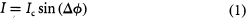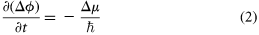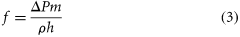Thank you for visiting nature.com. You are using a browser version with limited support for CSS. To obtain the best experience, we recommend you use a more up to date browser (or turn off compatibility mode in Internet Explorer). In the meantime, to ensure continued support, we are displaying the site without styles and JavaScript.

# Quantum oscillations between two weakly coupled reservoirs of superfluid 3He

## Abstract

Arguments first proposed over thirty years ago, based on fundamental quantum-mechanical principles, led to the prediction1,2,3 that if macroscopic quantum systems are weakly coupled together, particle currents should oscillate between the two systems. The conditions for these quantum oscillations to occur are that the two systems must both have a well defined quantum phase, φ, and a different average energy per particle, μ: the term ‘weakly coupled’ means that the wavefunctions describing the systems must overlap slightly. The frequency of the resulting oscillations is then given by f = (μ2− μ1)/h, where h is Planck's constant. To date, the only observed example of this phenomenon is the oscillation of electric current between two superconductors coupled by a Josephson tunnelling weak link4. Here we report the observation of oscillating mass currents between two reservoirs of superfluid 3He, the weak link being provided by an array of submicrometre apertures in a membrane separating the reservoirs. An applied pressure difference creates mass-current oscillations, which are detected as sound in a nearby microphone. The sound frequency (typically 6,000–200 Hz) is precisely proportional to the applied pressure difference, in accordance with the above equation. Thesesuperfluid quantum oscillations were first detected while monitoring an amplified microphone signal with the human ear.

## Main

The theory underlying the above equation was developed in the context of generalizing the ideas of Josephson4. He predicted that in a superconducting tunnel junction, the quantum phase difference, Δφ, across the function is related to the electrical current, I, through it, by the equationwhere Ic is the critical current of the junction. The phase difference evolves in time t according towhere is Planck's constant divided by 2π, and Δμ is the chemical-potential5 difference across the junction. For a fixed Δμ, Δφ evolves in time as Δφ = −Δμt/, and the corresponding current oscillates as I = Ic sin (−Δμt/). The chemical-potential difference (per Cooper pair) between two superconductors biased at voltage difference ΔV is given by Δμ = 2eΔV where e is the charge on the electron, and the corresponding Josephson frequency fj = 2eΔV/h = 4.82 × 1014 Hz V−1.

Equations (1) and (2), often called the d.c. and a.c. Josephson relations respectively, were re-derived in a more general context by Josephson, Anderson and Feynman1,2,3 who realized that the equations were applicable for any two, weakly coupled, phase-coherent systems. The dramatic aspect of the superconducting case is that the two coupled systems are macroscopic rather than microscopic.

For several decades physicists have recognized a close analogy between superconductors and superfluid helium6 in that they are both systems where a large number of particles occupy a single coherent quantum state. It has therefore been natural to search for quantum current oscillations in superfluids. The required conditions for the oscillations to exist in superfluids are that two samples of superfluid be separated by a region small enough so that the wavefunctions of each part can weakly penetrate and overlap that of the other. An example of this would be two reservoirs of superfluid helium in contact through a small aperture. Both the diameter and the length of the aperture must be comparable to the superfluid healing length7. This is the minimum length scale over which the magnitude of the superfluid wave function is allowed to vary for reasons of energy minimization. We refer to such an aperture as a weak link.

The equations which are the superfluid equivalent of the superconducting Josephson equations, have the same form as equations (1)and (2). The chemical-potential difference per particle on the right side of equation (2)becomes ΔPm/ρ. Here ΔP is the pressure difference across the weak link, ρ is the liquid density, and m is the mass of either the 4He atom or twice the 3He atomic mass, depending on whether one is describing 4He or 3He. (We are neglecting a small temperature term in the chemical potential because it is almost negligible in the experiments reported here.) In equation (1)the current, I, now refers to a mass current rather than an electric current. The pressure difference should therefore be accompanied by a mass supercurrent oscillating at the frequencywhich is 69 kHz Pa−1 for 4He, and 183.7 kHz Pa−1for 3He. We emphasize that this effect does not appear in ordinary fluids, or even for superfluids flowing through tubes which are not weak links. In the weak link, the fluid's response to an applied pressure difference is not simply to accelerate in proportion to that pressure gradient, but rather to oscillate in place at a frequency which is proportional to the pressure difference.

Demonstration of superfluid effects relying on these equations has been very elusive8. The fundamental problem is that a superfluid weak link requires an aperture whose dimensions are on the scale of the superfluid healing length, which is only 0.1 nm for 4He and 50 nm for 3He. To observe quantum oscillation phenomena one must first solve two technical problems; the fabrication of such small structures, and the measurement of the extremely small mass currents that would flow through such an aperture. Although these problems are not yet completely solved for 4He (ref. 9), recent advances have succeeded in both goals for 3He. Micro-fabrication techniques have led to the development of apertures which are submicrometre in all three dimensions10. At the same time, displacement transducer development11 (for gravity-wave detection) has led to technology that enables one to monitor the motion of a fluid-driving piston12, with sensitivity sufficient to detect the small currents predicted13,14,15,16,17,18 in a superfluid weak link. These technical advances have set the stage for observation of Josephson effects in 3He, and for study of the rich physical phenomena analogous to those observed in superconductors.

There is already one positive experimental indication19,20 of a 3He superfluid current–phase relation similar to equation (1). In that work, the superfluid 3He mass flow was through a single submicrometre aperture connected in parallel with a larger tube. The authors of references 19 and 20 report that, over a limited temperature range, they can numerically simulate the observed response of their nonlinear superfluid 3He oscillator, by using equations of motion that incorporate equations (1)and (2)into the dynamics of the system.

Encouraged by these reports, we have pursued the development of a 3He weak link21,22. Our device uses a square array (Fig. 1a) of 4,225 apertures spaced by 3,000 nm. Each aperture is 100 nm in diameter, in the same 50-nm-thick, silicon nitride membrane10. We use this 3He weak link in a temperature range where the superfluid healing length is greater than, or at least comparable to, the aperture diameter. We designed the array with the hope that the apertures would act coherently and have no interference between adjacent apertures, so that the array should behave like a single aperture but with 4,225 times greater current. One of the significant discoveries implied by the results reported below is the fact that the array does indeed behave in this desired manner.Figure 1: a, Scanning electron micrograph of a small area of the array of apertures in a silicon nitride (SiN) membrane whose thickness is 50.

The silicon chip containing the aperture array is placed in the wall of a pill-box-shaped cell, whose top and bottom surfaces are plastic membranes (Fig. 1b). The array is located in a lower, stiff membrane and the position of the upper, soft membrane is monitored with a sensitive displacement detector which can resolve motion as small as 10−14 m Hz−1/2. The upper membrane can be manipulated by the application of bias voltages between its conducting surface and a fixed electrode. The cell has an internal volume of 10 mm3, and takes several hours to fill by flow of normal liquid 3He through the array at temperature near 1 K. This cell is placed in a 3He-filled enclosure, which is in contact with a nuclear demagnetization refrigerator capable of cooling the 3He to temperatures well below its superfluid transition temperature (Tc = 0.929 mK).

We apply a step increase in bias potential to the soft membrane, which pulls it abruptly toward the fixed electrode. This creates a stepwise change in the pressure difference ΔP across the array, from zero initial pressure to a value determined by the applied voltage. We record the subsequent motion of the membrane's position, x(t), as mass flow through the array allows it to relax towards its new equilibrium position xf, where ΔP is again zero. The value of Δxxfx(t) is a measure of the instantaneous pressure across the aperture. If the aperture array behaves like a quantum weak link, the relaxing pressure difference ΔP(t) should be accompanied by mass-current oscillations within the aperture whose frequency is determined by equation (3).

A typical flow transient shows a relaxation of x(t) towards equilibrium. Owing to vibration noise in the displacement transducer, an oscilloscope trace (of the instantaneous voltage which is proportional to x) exhibits no remarkable structure suggestive of the predicted quantum oscillations. But if the electrical output of the displacement transducer is amplified and connected to audio headphones, the listener makes a most remarkable observation. As the pressure across the array relaxes to zero there is a clearly distinguishable tone smoothly drifting from high to low frequency during the transient, which lasts for several seconds. This simple observation marks the discovery of coherent quantum oscillations between weakly coupled superfluids.

To quantify this assertion we have processed several digitally recorded transient signals. Each transient is broken into equal, short time intervals. The average membrane position during the interval is used to calculate the pressure difference. For each time interval we compute the power spectrum of the membrane motion. As the frequency is drifting during any interval, the peak in the Fourier transform is not sharp. We identify the centre frequency of the strongest spectral component in the range of interest. This is the oscillation frequency corresponding to the pressure difference across the weak link during that interval.

Figure 2 plots the oscillation frequency, f, as a function of ΔP for a series of different temperatures. The frequency is found to be proportional to ΔP, the proportionality factor being 194 kHz Pa−1, with a statistical error of ±1 kHz Pa−1, and a systematic uncertainty of ±15 kHz Pa−1. The linear relationship between f and ΔP, and the measured value of the slope, are in clear agreement with equation (3), over a pressure range of more than two orders of magnitude. This is a direct confirmation of the quantum-oscillation equation for superfluid 3He.Figure 2: A graph of the frequency of the quantum oscillation detected at the top membrane as a function of ΔP, the pressure differen.

The relation between frequency and pressure difference is observed to be independent of temperature, to within the error of the measurement. It is expected13,14,17, that the actual current–phase relation might become increasingly distorted from the simple sine form in equation (1)as the coherence length gets smaller with falling temperature7. The distorted I (Δφ) would lead to higher harmonics of the quantum oscillations. We have searched for these higher harmonics in the spectral signal of the sound, and find that (to within our signal-to-noise ratio) they are absent over a wide range of temperatures.

The experimental results reported here lead to three significant conclusions. (1) The aperture array behaves like a single coherent superfluid weak link. (2) The current–phase relation is similar to the d.c. Josephson equation (equation (1)), that is periodic with modulus 2π. (3) The time evolution of the quantum phase across the array leads to current oscillations that drive the upper diaphragm at the frequency predicted by equation (3).

The remarkable properties of the weak-link array may lead to the observation of several physical phenomena analogous to those found in superconductivity. These include Shapiro23 steps and, perhaps most significantly, the development of the superfluid analogue of a d.c. SQUID24.

## References

1. 1

Josephson, B. D. Possible new effects in superconductive tunneling. Thesis, Cambridge Univ.((1962)).

2. 2

Anderson, P. W. in Lectures on the Many-Body Problem (ed. Caianello, E. R.) 113–135 (Academic, New York, (1964)).

3. 3

Feynman, R. P. The Feynman Lectures on Physics Sect. 21-9 (Addison Wesley, New York, (1965)).

4. 4

Josephson, B. D. Possible new effects in superconductive tunnelling. Phys. Lett. 1, 251–253 (1962).

5. 5

Reif, F. Fundamentals of Statistical and Thermal Physics Sect. 8-7 (McGraw-Hill, New York, (1965)).

6. 6

Tilley, D. R. & Tilley, J. Superfluidity and Superconductivity (Hilger, New York, (1990)).

7. 7

Vollhardt, D. & Woolfle, P. The Superfluid Phases of Helium-3 Sect. 7-2 (Taylor & Francis, New York, (1990)).

8. 8

Tilley, D. R. & Tilley, J. Superfluidity and Superconductivity Sect. 7-6 (Hilger, New York, (1990)).

9. 9

Pereverzev, S. V. Toward the Josephson effect in superfluid helium: how to make 20 nm holes in a 10 nm thick membrane. J. Low Temp. Phys. 101, 573–579 (1995).

10. 10

Amar, A., Sasaki, Y., Lozes, R. L., Davis, J. C. & Packard, R. E. Fabrication of submicron apertures in thin membranes of silicon nitride. J. Vac. Sci. Technol. B 11, 259–262 (1993).

11. 11

Paik, H. J. Superconducting tunable-diaphragm transducer for sensitive acceleration measurements J. Appl. Phys. 47, 1168–1178 (1976).

12. 12

Avenel, O. & Varoquaux, E. in Proc. 10th Int. Cryogenic Eng. Conf. (eds Klipping, G. & Klipping, I.) 587–589 (Butterworths, Guildford, (1986)).

13. 13

Monien, H. & Tewordt, L. Theory of Josephson flow oscillations in superfluid 3He-B. J. Low Temp. Phys. 62, 277–300 (1986).

14. 14

Hook, J. R. Current-phase relation for flow of an s-wave paired superfluid through a cylindrical channel of finite length. Jpn. J. Appl. Phys. 26, 159–160 (1987).

15. 15

Kurkijärvi, J. Josephson current through a short and narrow orifice in a p-wave superfluid. Phys. Rev. B 38, 11184–11187 (1988).

16. 16

Thuneberg, E. V. Two dimensional simulation of the Josephson Effect in superfluid 3He. Europhys. Lett. 7, 441–446 (1988).

17. 17

Ullah, S. & Fetter, A. L. Superfluid 3He-B in a weak link. Phys. Rev. B 39, 4186–4190 (1989).

18. 18

Thuneberg, E. V., Kurkijärvi, J. & Sauls, J. A. Quasiclassical theory of the Josephson effect in superfluid 3He. Physica B 165&166, 755–756 (1990).

19. 19

Avenel, O. & Varoquaux, E. Josephson effect and quantum phase slippage in superfluids. Phys. Rev. Lett. 60, 416–419 (1988).

20. 20

Varoquaux, E., Avenel, O., Ihas, G. & Salmelin, R. Phase slippage in superfluid 3He-B. Physica B 178, 309–316 (1990).

21. 21

Steinhauer, J. The current-pressure relation for a superfluid 3He weak link. Thesis, Univ. California, Berkeley((1995)).

22. 22

Mukharsky, Yu. M., Loshak, A., Schwab, K., Davis, J. C. & Packard, R. E. Study of an array of superfluid 3He weak links. Czech. J. Phys. 46, 115–116 (1996).

23. 23

Shapiro, S. Josephson currents in superconducting tunnelling; the effect of microwaves and other observations. Phys. Rev. Lett. 11, 80–82 (1963).

24. 24

Packard, R. E. & Vitale, S. Principles of superfluid-helium gyroscopes. Phys. Rev. B 46, 3540–3549 (1992).

## Acknowledgements

In early stages of this work we were helped by J. Steinhauer, K. Schwab, Yu. M. Murkarsky and I. Schuster. E. W. Hudson made important contributions to the data acquisition system. Figure 1b was made by R. Orr. The aperture array was constructed at the Berkeley Microfabrication Laboratory. This work was supported in part by the National Science Foundation, the Office of Naval Research, and the Packard Foundation.

## Author information

Authors

### Corresponding author

Correspondence to R. E. Packard.

## Supplementary information

### Supplementary Information

Supplementary material for the article (WAV 712 kb)

## Rights and permissions

Reprints and Permissions

Pereverzev, S., Loshak, A., Backhaus, S. et al. Quantum oscillations between two weakly coupled reservoirs of superfluid 3He. Nature 388, 449–451 (1997). https://doi.org/10.1038/41277

• Accepted:

• Issue Date:

• ### Periodic squeezing in a polariton Josephson junction

• , Mitchell D. Anderson
• , Hugo Flayac
• , Marcia T. Portella-Oberli
• , Fauzia Jabeen
• , Claudéric Ouellet-Plamondon
• , Gregory C. Sallen
•  & Benoit Deveaud

Nature Communications (2017)

• ### Polaritonic Rabi and Josephson Oscillations

• Amir Rahmani
•  & Fabrice P. Laussy

Scientific Reports (2016)

• ### Polariton condensation in solitonic gap states in a one-dimensional periodic potential

• D. Tanese
• , H. Flayac
• , D. Solnyshkov
• , A. Amo
• , A. Lemaître
• , E. Galopin
• , R. Braive
• , P. Senellart
• , I. Sagnes
• , G. Malpuech
•  & J. Bloch

Nature Communications (2013)

• ### Order-related acoustic characterization of production data

• M. Iber
•  & K. Windt

Logistics Research (2012)

• ### Generalized Rotational Susceptibility Studies of Solid 4He

• , E. J. Pratt
• , B. Hunt
• , M. Yamashita
• , M. J. Graf
• , A. V. Balatsky
•  & J. C. Davis

Journal of Low Temperature Physics (2012)